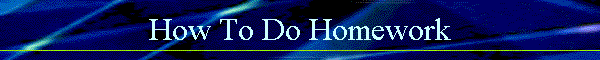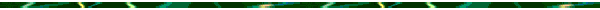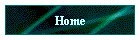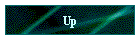HOW TO DO YOUR ALGEBRA 2 ASSIGNMENTS     Setting up your paper: 1) All work must be done in pencil! 2) Use only 8 1/2" by 11" white lined paper. 3) Put a complete heading on the first page of all assignments in the upper left-hand corner of your paper. Include your name, name of class, period, and date. 4) Write the description of the complete assignment on the first line of the paper (page number(s) and problem numbers). 5) Skip one line after the heading and assignment name. 6) Put the assignment number in a circle in the top right corner of the first page. 7) Make an answer column (optional, see #11 below) with a ruler from the top to the bottom of the page.  Number each line of the answer column to the left of the answer column line.   Doing the Work: 8) The first step of a problem is always to copy down the original problem. 9 ) All required steps must be shown!!! 10 ) Each step of a problem must be on one line. The next step must be under   the last step. 11 ) The last line of the problem is your answer. Circle or highlight your answer.  You can either circle or highlight your answer or write down this answer again in the answer column (if you circle your answers, you do not need an answer column). Put "see work" or "see graph" in the answer column where appropriate. 12 ) Number all problems and work across your paper from left to right. 13) Skip one line between one row of problems and the next row.   Correcting and handing it in: 14) Keep all the assignments in your notebook in reverse numerical order (in descending order or highest numbered assignment to lowest). 15) Correct your assignments with pen (any color pen will do, but do not use colored pencils or an erasable pen). Remember that every incorrect problem needs to be completely rewritten correctly. 16 ) Write your score as a fraction at the top of the first page of each assignment:                                                   number correct                                            number of problems